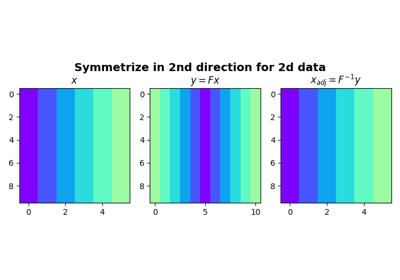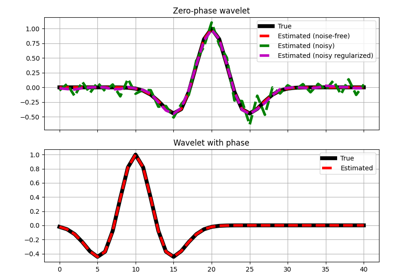# pylops.Symmetrize#

class pylops.Symmetrize(dims, axis=-1, dtype='float64', name='S')[source]#

Symmetrize along an axis.

Symmetrize a multi-dimensional array along axis.

Parameters
dims

Number of samples for each dimension (None if only one dimension is available)

axisint, optional

New in version 2.0.0.

Axis along which model is symmetrized.

dtypestr, optional

Type of elements in input array

namestr, optional

New in version 2.0.0.

Name of operator (to be used by pylops.utils.describe.describe)

Notes

The Symmetrize operator constructs a symmetric array given an input model in forward mode, by pre-pending the input model in reversed order.

For simplicity, given a one dimensional array, the forward operation can be expressed as:

$\begin{split}y[i] = \begin{cases} x[i-N+1],& i\geq N\\ x[N-1-i],& \text{otherwise} \end{cases}\end{split}$

for $$i=0,1,2,\ldots,2N-2$$, where $$N$$ is the dimension of the input model.

In adjoint mode, the Symmetrize operator assigns the sums of the elements in position $$N-1-i$$ and $$N-1+i$$ to position $$i$$ as follows:

$\begin{multline} x[i] = y[N-1-i]+y[N-1+i] \quad \forall i=0,2,\ldots,N-1 \end{multline}$

apart from the central sample where $$x = y[N-1]$$.

Attributes
shapetuple

Operator shape

explicitbool

Operator contains a matrix that can be solved explicitly (True) or not (False)

Methods

 __init__(dims[, axis, dtype, name]) adjoint() apply_columns(cols) Apply subset of columns of operator cond([uselobpcg]) Condition number of linear operator. conj() Complex conjugate operator div(y[, niter, densesolver]) Solve the linear problem $$\mathbf{y}=\mathbf{A}\mathbf{x}$$. dot(x) Matrix-matrix or matrix-vector multiplication. eigs([neigs, symmetric, niter, uselobpcg]) Most significant eigenvalues of linear operator. matmat(X) Matrix-matrix multiplication. matvec(x) Matrix-vector multiplication. reset_count() Reset counters rmatmat(X) Matrix-matrix multiplication. rmatvec(x) Adjoint matrix-vector multiplication. todense([backend]) Return dense matrix. toimag([forw, adj]) Imag operator toreal([forw, adj]) Real operator tosparse() Return sparse matrix. trace([neval, method, backend]) Trace of linear operator. transpose()

## Examples using pylops.Symmetrize#Symmetrize

SymmetrizeWavelet estimation

Wavelet estimation